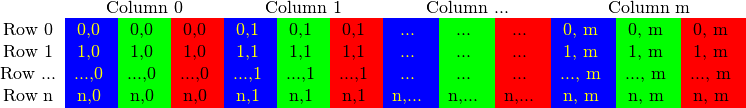# OpenCV学习笔记

2020/02/02

OpenCV的学习笔记整理，包括的都是一些常见用法，例如Mat的遍历等。

## cv::Mat Usage 使用说明

### Mat构成

cv::Mat的构成很特殊。它包含两个部分：

• 一个指向矩阵主体数据的指针。也就是说，两个不同的cv::Mat的指针可以指向同一块数据区域。这样的好处是，图片通常都比较大，这样无需分配额外内存来存储多余的图片。当然缺点很明显，如果Mat指向的数据区域被release的话，所有的指向它的Mat都会失效。这样的话，将来再次尝试获取某个像素的话就会出错。

``````// 此时只是定义了矩阵的header部分，还未给矩阵主体分配空间
cv::Mat A, C;
// 读入图片同时为矩阵主体分配内存空间
// 此后如果修改B的话，A指向的内容也会被改变，这很危险。
cv::Mat B(A);
C = A;
``````

``````Mat F = A.clone(); // 直接硬拷贝
Mat G;
A.copyTo(G); // copyTo和clone()结果完全一样，就是用法稍有不同
``````

### 初始化

``````// 初始化元素完全相同
Mat M(2,2, CV_8UC3, Scalar(0,0,255)); // 最后一个参数是初始化的constant数值
// 初始化元素不相同
cv::Mat T1 = (cv::Mat_<double> (3,4) <<
1,0,0,0,
0,1,0,0,
0,0,1,0); // cv::Mat_是最底层的Mat类，需要重载元素类型。注意这种初始化方式是行优先的（row-major）
``````

### Mat成员函数

``````if (img.depth() == CV_8U){
// depth()函数获取的是深度，即每个像素点中每个元素的类型。这里就是8-bit unsigned char类型
}
if (img.type() == CV_8UC3){
// type()函数获取的是详细类型，这里就是8-bit unsigned char且有3个channels
}
if (img.channels() == 3){
// channels()获取的是每个像素点的元素个数，例如如果是BGR图片，这个值就是3
}
``````

### Mat的类型

Mat的类型可以通过成员函数`type()`获取，但是它输出的是一个整数而不是可读性好的类似8UC1这种。例如，0就对应8UC1, 18是8UC3等。并且，OpenCV中居然没有将前者转换成后者的函数。下面给出这个函数，并且注释中有输出的整数和类型的对照表。

``````//! Convert the type of a cv::Mat object to a readable string
/*
* Correspondence between type number and string is in this table:
*
+--------+----+----+----+----+------+------+------+------+
|        | C1 | C2 | C3 | C4 | C(5) | C(6) | C(7) | C(8) |
+--------+----+----+----+----+------+------+------+------+
| CV_8U  |  0 |  8 | 16 | 24 |   32 |   40 |   48 |   56 |
| CV_8S  |  1 |  9 | 17 | 25 |   33 |   41 |   49 |   57 |
| CV_16U |  2 | 10 | 18 | 26 |   34 |   42 |   50 |   58 |
| CV_16S |  3 | 11 | 19 | 27 |   35 |   43 |   51 |   59 |
| CV_32S |  4 | 12 | 20 | 28 |   36 |   44 |   52 |   60 |
| CV_32F |  5 | 13 | 21 | 29 |   37 |   45 |   53 |   61 |
| CV_64F |  6 | 14 | 22 | 30 |   38 |   46 |   54 |   62 |
+--------+----+----+----+----+------+------+------+------+
*/
std::string getCvMatType(int type)
{
std::string r;

uchar depth = type & CV_MAT_DEPTH_MASK;
uchar chans = 1 + (type >> CV_CN_SHIFT);

switch (depth)
{
case CV_8U:
r = "8U";
break;
case CV_8S:
r = "8S";
break;
case CV_16U:
r = "16U";
break;
case CV_16S:
r = "16S";
break;
case CV_32S:
r = "32S";
break;
case CV_32F:
r = "32F";
break;
case CV_64F:
r = "64F";
break;
default:
r = "User";
break;
}
r += "C";
r += (chans + '0');
return r;
}
``````

### 其它使用方法

``````// 获取一个Mat中的一小块矩形部分
cv::Mat small_img = img(cv::Rect(0, 0, 100, 200)); // 注意这里依然是只拷贝了header
// 修改这个小块。注意此时img指向的矩阵主体也会改变，因为它们指向同一个主体部分
small_img.setTo(0);
``````

## Access an image 操作图片像素

OpenCV中，一张图片中每个像素点可能只有一个unsigned char变量，即8-bit元素，例如灰度图片；也可能是多个unsigned char变量，例如RGB或者RGBA彩色图片。这里每个像素中有几个量就叫做通道（channels）。灰度图片（grayscale）就是单通道，RGB图片就是三通道。下图演示了RGB图片中的数据存储：• OpenCV中读入彩色图片到`cv::Mat`中后，默认是按照BGR顺序，即蓝绿红，如上图所示。而通常很多图片存储后是按照RGB顺序的。这点一定要注意。
• 可以通过`cv::Mat`类的成员函数`channels()`获取该图片的通道个数。

### Get one pixel 获取一个像素

``````// 灰度图像每个像素点就是一个unsigne char
unsigned char gray = gray_img.at<uchar>(i,j);
// 彩色图像每个像素点包含RGB这三个channels（有时候是RGBA四个）
unsigned char b = color_img.at<cv::Vec3b>(i,j);
unsigned char g = color_img.at<cv::Vec3b>(i,j);
unsigned char r = color_img.at<cv::Vec3b>(i,j);
``````

### Scan entire image 遍历整张图片

#### 1. The efficient way 最快的方法：基于C指针

``````// 遍历整张图片，并将每个像素的灰度值通过table来in-place得映射到另一个值
Mat& ScanImageAndReduceC(Mat& I, const uchar* const table)
{
// accept only char type matrices
CV_Assert(I.depth() == CV_8U);
int channels = I.channels();
int nRows = I.rows;
int nCols = I.cols * channels;
// 检查一下是否是连续存储的。如果是，则更新行和列的值。
if (I.isContinuous())
{
nCols *= nRows;
nRows = 1;
}
int i,j;
uchar* p;
for( i = 0; i < nRows; ++i)
{
p = I.ptr<uchar>(i); // 先获取每一行开头的指针
for ( j = 0; j < nCols; ++j)
{
// p[j]就是每个像素了。
// Here it maps each pixel's grayscale value to another value.
p[j] = table[p[j]];
}
}
return I;
}
``````

### 2. The iterator (safe) way 迭代器做法（更安全）

``````// 依然是遍历整张图片，并将每个像素的每个通道的颜色值通过table来in-place得映射到另一个值
Mat& ScanImageAndReduceIterator(Mat& I, const uchar* const table)
{
// accept only char type matrices
CV_Assert(I.depth() == CV_8U);
const int channels = I.channels();
switch(channels)
{
case 1:
{
MatIterator_<uchar> it, end;
for( it = I.begin<uchar>(), end = I.end<uchar>(); it != end; ++it)
*it = table[*it];
break;
}
case 3:
{
MatIterator_<Vec3b> it, end;
for( it = I.begin<Vec3b>(), end = I.end<Vec3b>(); it != end; ++it)
{
(*it) = table[(*it)];
(*it) = table[(*it)];
(*it) = table[(*it)];
}
}
}
return I;
}
``````

#### 3. On-the-fly address calculation 即时计算每个像素的地址

``````Mat& ScanImageAndReduceRandomAccess(Mat& I, const uchar* const table)
{
// accept only char type matrices
CV_Assert(I.depth() == CV_8U);
const int channels = I.channels();
switch(channels)
{
case 1:
{
for( int i = 0; i < I.rows; ++i)
for( int j = 0; j < I.cols; ++j )
I.at<uchar>(i,j) = table[I.at<uchar>(i,j)]; // 和前一种方法唯一的不同之处
break;
}
case 3:
{
// 下面使用了Mat_类型，它是一种Mat的指针类型。使用它的好处是，在循环中就不用每次都要显式的像I.at<Vec3b>这样指定类型了。当然它的速度其实是和直接使用.at<Vec3b>一模一样，因此它的好处只是让代码更简洁一点而已。
Mat_<Vec3b> _I = I;
for( int i = 0; i < I.rows; ++i)
for( int j = 0; j < I.cols; ++j )
{
_I(i,j) = table[_I(i,j)];
_I(i,j) = table[_I(i,j)];
_I(i,j) = table[_I(i,j)];
}
I = _I;
break;
}
}

return I;
}
``````

#### 4. The Core Function 直接使用OpenCV相关函数

``````Mat& ScanImageAndReduceCoreFunction(Mat& I, const uchar* const table)
{
// 定义look-up table变量是一个1x256的矩阵
Mat lookUpTable(1, 256, CV_8U);
uchar* p = lookUpTable.data;
for( int i = 0; i < 256; ++i)
p[i] = table[i]; // 因为lookUpTable只有一行，因此直接赋值即可
cv::Mat J = I.clone();
cv::LUT(I, lookUpTable, J); // 调用已定义的函数，J是输出
return J;
}
``````

## 一些常见的函数

``````cv::Mat mask;
cv::Mat E = cv::findEssentialMat(selected_points1, selected_points2, Kd.at<double>(0,0), cv::Point2I d(image1.cols/2., image1.rows/2.),
vector<cv::Point2f> inlier_match_points1, inlier_match_points2;
for(int i = 0; i < mask.rows; i++) {
// 只对选中的特征点进行操作
inlier_match_points1.push_back(selected_points1[i]);
inlier_match_points2.push_back(selected_points2[i]);
}
}
``````

### 常见的 cv::Mat 的操作

• 两个 Mat 之间的加减乘除
• 和另一个 Mat 的位操作，例如与或非；
• 一些特殊的计算，例如指数对数；
• 特征值特征向量；
• 求和、平方和、最大值、最小值、方差、均值、直方图等各种统计；